Next: Coupled rearrangement channel method Up: Variational approaches Previous: Variational approaches

### Hylleraas type basis expansion

A popular choice are Hylleraas type functions [106,107,108,109], which for J=0 states read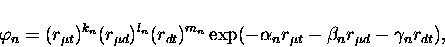(34)

and are expressed in the interparticle co-ordinates, where rxy denotes the distance between the particle x and y. The exponential parameters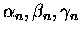are often taken to be the same for all n to reduce the number of parameters to be optimized. For loosely bound states (1,1) of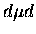and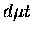, similar functions with ki=li=mi, known as Slater gemials were found to be more useful in representing the large physical size of the states [110,84]. The disadvantage with Slater gemials is that one has to optimize a large number of exponential parameters, which is very time consuming. Another disadvantage is its linear dependencies'' problem. This is due to the fact that the basis in this set is nearly linearly dependent, i.e. rather non-orthogonal. Since the functions differ only by their exponents, an optimized basis set sometimes has several functions with close values of the exponents . Thus, the use of extended arithmetic precision (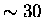decimal digits) is necessary.Next: Coupled rearrangement channel method Up: Variational approaches Previous: Variational approaches